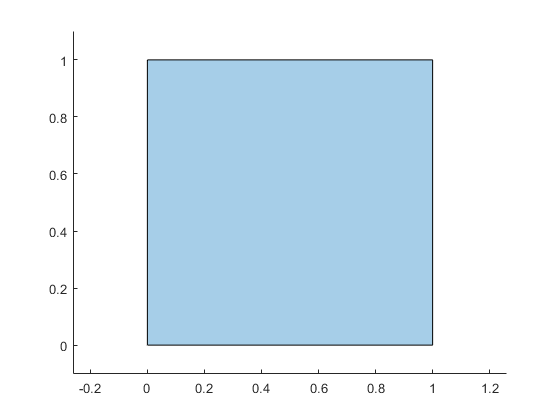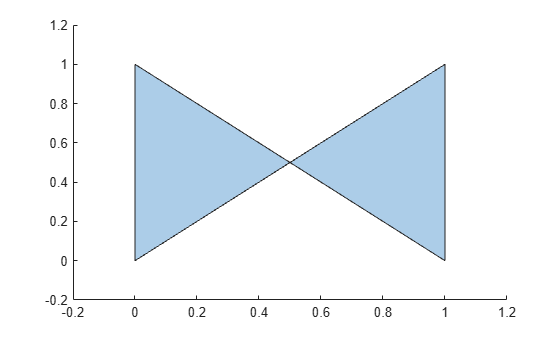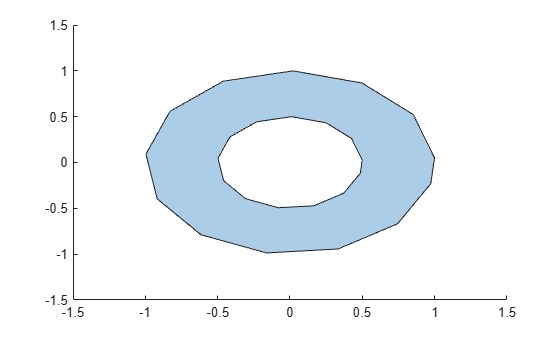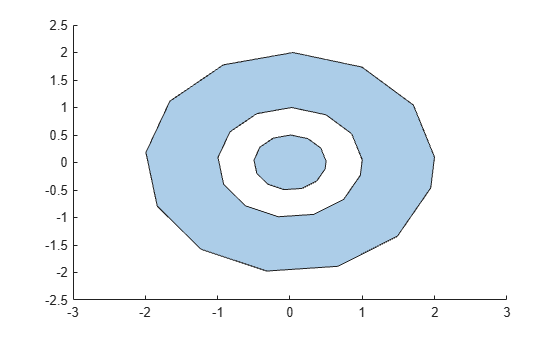# polyshape

2-D polygons

## Description

The `polyshape` function creates a polygon defined by 2-D vertices, and returns a `polyshape` object with properties describing its vertices, solid regions, and holes. For example, ```pgon = polyshape([0 0 1 1],[1 0 0 1])``` creates the solid square defined by the four points (0,1), (0,0), (1,0), and (1,1).

`>> pgon = polyshape([0 0 1 1],[1 0 0 1])`
```pgon = polyshape with properties: Vertices: [4×2 double] NumRegions: 1 NumHoles: 0```
`>> plot(pgon)`## Creation

When creating a `polyshape` object, the input vertices should define one or more boundaries of a polygon that have no intersections and are properly nested. Otherwise, the `polyshape` function automatically alters the input vertices as needed to create a well-defined polygon.

If you want to create a regular polygon, you can also use the `nsidedpoly` function. `nsidedpoly` enables you to optionally specify parameters such as the center point and side length of the polygon.

### Syntax

``pgon = polyshape()``
``pgon = polyshape(x,y)``
``pgon = polyshape(P)``
``pgon = polyshape(X,Y)``
``pgon = polyshape(___,Name,Value)``

### Description

````pgon = polyshape()` creates an empty `polyshape` object.```

example

````pgon = polyshape(x,y)` creates a `polyshape` from 2-D vertices defined by a vector of x-coordinates and a vector of corresponding y-coordinates. `x` and `y` must be the same length with at least three elements.```

example

````pgon = polyshape(P)` creates a `polyshape` from the 2-D vertices defined in the N-by-2 matrix `P`, where N is the number of vertices. The first column of `P` defines the x-coordinates, and the second column defines the `y`-coordinates.```

example

````pgon = polyshape(X,Y)`, where `X` and `Y` are 1-by-M cell arrays of vectors for the x- and `y`-coordinates, creates a polygon consisting of M boundaries. Each vector in `X` must have the same length as the corresponding vector in `Y`, but the number of vertices can vary between boundaries.```

example

````pgon = polyshape(___,Name,Value)` specifies additional parameters for creating a `polyshape` object for any of the previous syntaxes.```

### Input Arguments

expand all

x-coordinates of polygon vertices, specified as a vector. You can represent the coordinates of multiple boundaries at a time by placing a `NaN` between each boundary. For example, ```pgon = polyshape([0 0 1 NaN 1 5 5],[1 0 0 NaN 5 5 1])``` creates a `polyshape` made up of two solid triangles.

Numeric input coordinates that are not of type `double` are automatically converted to type `double`.

Data Types: `double` | `single` | `int8` | `int16` | `int32` | `int64` | `uint8` | `uint16` | `uint32` | `uint64`

y-coordinates of polygon vertices, specified as a vector. You can represent the coordinates of multiple boundaries at a time by placing a `NaN` between each boundary. For example, ```pgon = polyshape([0 0 1 NaN 1 5 5],[1 0 0 NaN 5 5 1])``` creates a `polyshape` made up of two solid triangles.

Numeric input coordinates that are not of type `double` are automatically converted to type `double`.

Data Types: `double` | `single` | `int8` | `int16` | `int32` | `int64` | `uint8` | `uint16` | `uint32` | `uint64`

2-D vertices of the polygon, specified as a 2-column matrix. The first column of `P` contains the x-coordinates of the vertices, and the second column contains the y-coordinates. `P` must have at least 3 rows.

You can represent the coordinates of multiple boundaries at a time by placing a `NaN` between each boundary. For example, ```pgon = polyshape([1 0; 0 0; 0 1; NaN NaN; 1 5; 5 5; 5 1])``` creates a `polyshape` made up of two solid triangles.

Numeric input coordinates that are not of type `double` are automatically converted to type `double`.

Data Types: `double` | `single` | `int8` | `int16` | `int32` | `int64` | `uint8` | `uint16` | `uint32` | `uint64`

x-coordinates of M boundaries, specified as a cell array of vectors. The length of each vector can vary, but must match the length of the corresponding y vector.

Numeric input coordinates that are not of type `double` are automatically converted to type `double`.

Data Types: `cell`

y-coordinates of M boundaries, specified as a cell array of vectors. The length of each vector can vary, but must match the length of the corresponding y vector.

Numeric input coordinates that are not of type `double` are automatically converted to type `double`.

Data Types: `cell`

Name-Value Arguments

Specify optional pairs of arguments as `Name1=Value1,...,NameN=ValueN`, where `Name` is the argument name and `Value` is the corresponding value. Name-value arguments must appear after other arguments, but the order of the pairs does not matter.

Before R2021a, use commas to separate each name and value, and enclose `Name` in quotes.

Example: ```pgon = polyshape(P,'SolidBoundaryOrientation','ccw')```

Boundary orientation, specified as the comma-separated pair consisting of `'SolidBoundaryOrientation'` and one of these values:

• `'auto'` — Automatically determine the boundary type based on boundary nesting.

• `'cw'` — Treat clockwise vertex orientation as a solid boundary.

• `'ccw'` — Treat counterclockwise vertex orientation as a solid boundary.

This parameter is typically specified for consistency purposes when polygon data is produced by other software using a particular convention.

Vertex alteration, specified as the comma-separated pair consisting of `'Simplify'` and one of the following:

• `true` — Alter polygon vertices to produce a well-defined polygon when the input vertices produce intersections or improper nesting.

• `false` — Do not alter input vertices regardless of intersections or improper nesting. Computing with ill-defined polygons can lead to inaccurate or unexpected results.

Data Types: `logical`

Collinear vertices, specified as the comma-separated pair consisting of `'KeepCollinearPoints'` and one of the following:

• `false` — Remove collinear points when creating the `polyshape` so that it contains the fewest vertices necessary to define the boundaries.

• `true` — Keep all collinear points as vertices when creating the `polyshape`.

After creating a `polyshape`, the value of `'KeepCollinearPoints'` is automatically carried over when you use the `addboundary` or `simplify` object functions.

Data Types: `logical`

## Properties

expand all

2-D vertices of the polygon, specified as a 2-column matrix. The vertex matrix contains the vertices for each boundary of the polygon. `NaN` values separate each boundary's set of vertices.

Data Types: `double`

Number of regions making up the polygon, specified as a scalar integer. A region is an area bounded by an outer boundary, which may contain hole boundaries that lie entirely inside the outer boundary.

Data Types: `double`

Number of holes in the polygon, specified as a scalar integer.

Data Types: `double`

## Object Functions

expand all

 `addboundary` Add `polyshape` boundary `polybuffer` Create buffer around points, lines, or `polyshape` objects `rmboundary` Remove `polyshape` boundary `rmholes` Remove holes in `polyshape` `rmslivers` Remove `polyshape` boundary outliers `rotate` Rotate `polyshape` `scale` Scale `polyshape` `simplify` Simplify `polyshape` boundaries `sortboundaries` Sort `polyshape` boundaries `sortregions` Sort `polyshape` regions `translate` Translate `polyshape`
 `boundary` Vertex coordinates of `polyshape` boundary `holes` Convert `polyshape` hole boundaries to array of `polyshape` objects `ishole` Determine if `polyshape` boundary is a hole `isinterior` Query points inside `polyshape` `issimplified` Determine if `polyshape` is well-defined `nearestvertex` Query nearest `polyshape` vertex `numboundaries` Number of `polyshape` boundaries `numsides` Number of `polyshape` sides `overlaps` Determine whether `polyshape` objects overlap `plot` Plot `polyshape` `regions` Access `polyshape` regions
 `area` Area of `polyshape` `boundingbox` Bounding box of `polyshape` `centroid` Centroid of `polyshape` `convhull` Convex hull of `polyshape` `perimeter` Perimeter of `polyshape` `triangulation` Triangulate `polyshape` `turningdist` Compute turning distance between `polyshape` objects
 `intersect` Intersection of `polyshape` objects `subtract` Difference of two `polyshape` objects `union` Union of `polyshape` objects `xor` Exclusive OR of two `polyshape` objects

## Examples

collapse all

Create and plot a polygon made up of four points, and compute its area, perimeter, and centroid coordinates.

```pgon = polyshape([0 0 1 3], [0 3 3 0]); plot(pgon)````A = area(pgon)`
```A = 6 ```
`P = perimeter(pgon)`
```P = 10.6056 ```
`[Cx Cy] = centroid(pgon)`
```Cx = 1.0833 ```
```Cy = 1.2500 ```

Analyze a polygon with intersecting boundaries.

Create a polygon whose boundary contains a self-intersection. By default, the `polyshape` function splits the boundary into two distinct boundaries in order to create a well-defined polygon.

```P = [0 0; 1 1; 1 0; 0 1; 0 0]; pgon = polyshape(P)```
```Warning: Polyshape has duplicate vertices, intersections, or other inconsistencies that may produce inaccurate or unexpected results. Input data has been modified to create a well-defined polyshape. ```
```pgon = polyshape with properties: Vertices: [7x2 double] NumRegions: 2 NumHoles: 0 ```

Plot the polygon and compute its area.

`plot(pgon)``A = area(pgon)`
```A = 0.5000 ```

If you do not simplify the original input vertices, the areas of the two triangular regions of the polygon cancel each other out.

`pgon2 = polyshape(P,'Simplify',false)`
```pgon2 = polyshape with properties: Vertices: [4x2 double] NumRegions: 1 NumHoles: 0 ```
`A2 = area(pgon2)`
```A2 = 0 ```

Analyze polygons with nested boundaries.

Create a polygon with two properly nested boundaries. `polyshape` defines the outermost boundary as the exterior bound of a solid region. Working inward, the next boundary defines the start of a hole.

```t = 0.05:0.5:2*pi; x1 = cos(t); y1 = sin(t); x2 = 0.5*cos(t); y2 = 0.5*sin(t); pgon = polyshape({x1,x2},{y1,y2})```
```pgon = polyshape with properties: Vertices: [27x2 double] NumRegions: 1 NumHoles: 1 ```
`plot(pgon)`Use the `addboundary` function to create a second polygon that adds a third, outer boundary to `pgon`.

```x3 = 2*cos(t); y3 = 2*sin(t); pgon2 = addboundary(pgon,x3,y3)```
```pgon2 = polyshape with properties: Vertices: [41x2 double] NumRegions: 2 NumHoles: 1 ```
`plot(pgon2)`Adding a third, outer boundary requires `polyshape` to reorganize the solid and hole boundaries based on the new nesting pattern. Again, `polyshape` starts with the outermost boundary that indicates the start of a solid region, then alternates between hole and solid with each nested boundary, working inward. The new polygon now has two solid regions and one hole.

## Version History

Introduced in R2017b